# Digging Deeper into … Number Theory (5th & 6th classes)

## Digging Deeper into … Number Theory (5th & 6th classes)

For practical suggestions for families, and links to useful digital resources, to support children learning about the topic of number theory, please check out the following post: Dear Family, your Operation Maths Guide to Number Theory

Number Theory is a number topic that is concerned with subcategories of whole numbers: odd and even; factors and multiples; prime and composite; square, triangular and rectangular numbers; square roots and exponential numbers.

While 5th class is the first time that the children formally meet this strand unit, they have engaged with elements of the topic in previous classes:

• odd and even numbers were explored formally in 1st, 2nd and 3rd classes
• the language of factors and multiples was used in 3rd, 4th and 5th classes as part of the multiplication and division chapters.

### Concrete-pictorial-abstract approach (CPA)

Traditionally this topic is often taught in a quite abstract way e.g. using operations to calculate square numbers, square roots identifying prime numbers by the number of  factors, etc. However, number theory by its nature, prompts us to consider and explore the shape and arrangements of numbers and, thus, is ideal to be explored in a visual way. Even Lego can be used to explore square numbers, arrays and factors!

Therefore, the activities will once again follow a concrete-pictorial-abstract approach, as is used throughout Operation Maths with the emphasis being on the children being able to build and/or visualise the shape of the numbers themselves, before progressing to using more abstract means to identify larger numbers, compare patterns, etc.Therefore, the focus in this topic should not be on the numerals themselves, rather on the shape of the numbers; it is one thing to recognise or identify odd and even numbers, factors, prime, composite, squared numbers, cubed numbers, etc., it’s another thing to visualise them. Using concrete materials or pictorial representations is vital for the children to really develop their number sense and their appreciation of these numbers and how they relate to,  and interact with, each other.

### Number Theory in the real world

This topic is very interesting in the way it relates to spatial arrangements in real life. Triangular numbers can be seen in arrangements of bowling skittles, snooker balls, displays in the supermarket. Even numbers are evident wherever there is a pair.Encourage the children to suggest examples and applications of odd and even numbers, prime and composite numbers, squared numbers, cubed numbers, etc. in the world around them. The idea of videos going viral on the internet, rumours or secrets being spread can all be related back to exponential numbers.

And as the children explore more, they may also begin to discover other fascinating connections between various types of numbers such as the total of two consecutive triangular numbers is always a square number and that double any triangular number is always a rectangular number. Again the emphasis should be on the children discovering this for themselves through engagement in rich tasks, such as the one shown below from the Early Finishers photocopiable in the TRB.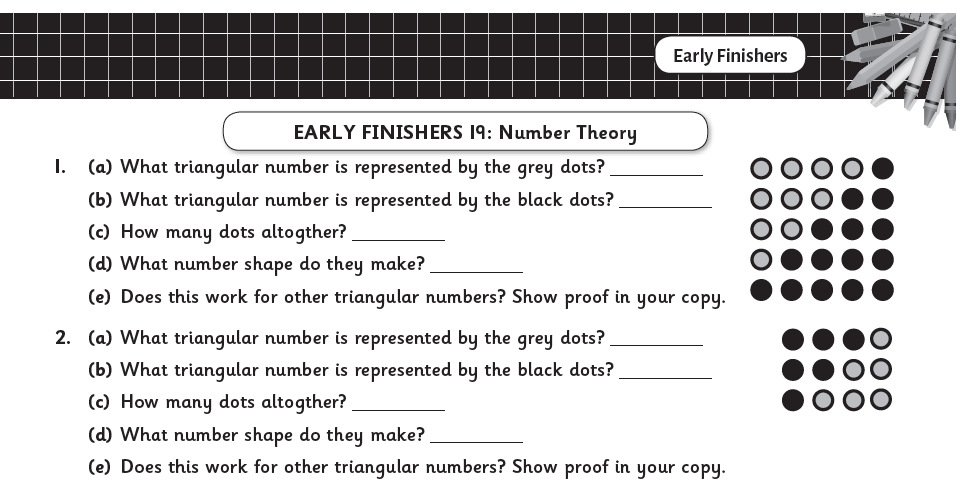### Points to note for the teacher

• Counting/Natural numbers: When we talk about numbers in this topic we are referring to the set of counting numbers (i.e. 1, 2, 3, 4 … ) or natural numbers. These are whole numbers (no fractions or decimals) and do not include negative numbers. Since there is no universal agreement about whether to include zero in the set of natural numbers (some define the natural numbers to be the positive integers {1, 2, 3, …}, while for others the term designates the non-negative integers {0, 1, 2, 3, …}) in Operation Maths we only consider the positive integers, i.e. not including zero.
• That which constitutes a rectangular number is undefined in the mathematics curriculum and the definition varies from source to source, with many conflicting with each other, particularly online. In Operation Maths, we have gone with the definition, as shown in the image below, given in the NCCA Bridging Glossary. This defines rectangular numbers as n x (n+1) which can also be expressed as n² + n; thus, the 6th rectangular number is 6 x 7 or 6² + 6 both of which equal 42.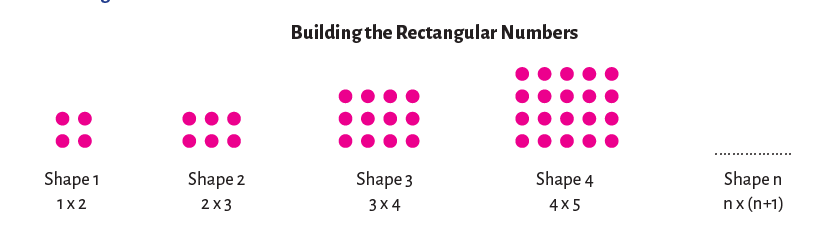• Only whole numbers can be even or odd. The children may think that 1.2 or 4.36 are even numbers since their last digits is one of 0, 2, 4, 6, 8. However, only whole numbers can be denoted even or odd; you cannot create either an even or odd cube pattern (as shown in the Operation Maths 5 Pupils’ Book, p. 153), using 1 and a bit cubes.
• Factors V multiplicands. In this sentence, 1.2 × 1.5 = 1.8, 1.2 and 1.5 are strictly speaking not factors even though they are being multiplied to produce a product. This is because factors are whole numbers, and when we ask for the factors of a particular number we want the whole number divisors of that number; if we were to include fractions (or decimal fractions) then the list would be impossibly long, complex and almost infinite. In the number sentence above, 1.2 and 1.5 are multiplicands or can also be referred to as decimal factors.
• Factor pairs should be listed in a systematic way so as to identify all the pairs, i.e. not omit any, or equally not repeat pairs. Many children forget to include 1 and its pair as a factor, or any factors that are outside the traditional limits of the 10 × 10 factors,
e.g. omitting 1 × 36, or 2 × 18 as factors of 36. The may also rewrite a pair, e.g. (2,3) and (3,2), not appreciating that because of the commutative property, order doesn’t matter and this is the same pair. To avoid these mistakes, demonstrate to the children how to identify factor pairs in a systematic way using a T-chart, as shown below.• Dear Family, your Operation Maths Guide to Number Theory includes practical suggestions for supporting children, and links to a huge suite of digital resources.
• Virtual Maths Manipulatives for Algebra: Lots of tools that can be used in many different ways to explore odd and even numbers, factors, multiples, prime and composite numbers etc.
• For more hints and tips specific to each class level, check out the “What to look out for” section in the introduction to this topic in the Teacher’s Resource Book (TRB)
• Operation Maths Digital Resources: As always don’t forget to access the linked digital activities on the digital version of the Pupil’s book, available on edcolearning.ie. Tip: look at the footer on the first page of each chapter in the pupil’s book to get a synopsis of what digital resources are available/suggested to use with that particular chapter.
• Explore exponential numbers via these lessons on the spreading of rumours and secrets.
• Using Lego to develop math concepts: Read this article to discover ways to use Lego to explore square numbers, arrays and factors
• NRICH: selection of problems, articles and games for prime numbers.
• Check out this Number Theory Board on Pinterest

## Digging Deeper into … Multiplication and Division (3rd – 6th classes)

Category : Uncategorized

For practical suggestions for families, and links to useful digital resources, to support children learning about the topic of multiplication and division, please check out the following post: Dear Family, your Operation Maths Guide to Multiplication and Division

Children are formally introduced to the operations of multiplication and division in third class, although their initial exploration of these concepts would have begun in 1st and 2nd classes with basic skip counting in jumps of 2s, 10s, 5s and, to a lesser extent, 3s and 4s. Thus, most children become quite comfortable with the number facts of twos, fives and tens quite quickly because these facts are more familiar to them. That said, when starting multiplication and division, and particularly when doing these groups of “easier” facts, it is still very important that the children are given ample time and experiences to develop a solid understanding of the concepts of multiplication and division themselves. This is why this topic is typically taught as a double (i.e. two week) chapter in Operation Maths.

Furthermore, as with the Operation Maths approach to addition and subtraction, multiplication and division is, for the most part, taught together, so as to reinforce them as related concepts that are also the inverse of each other. Thus, the initial activities in the Discovery Book, often require the children to reflect on their understanding of the concepts and to compare and contrast them.

As the children move through the classes, it is anticipated that they would begin to use this understanding of multiplication and division as the inverse of each other, to recognise the quotient (answer) in division as a missing factor in the inverse multiplication fact, e.g. asking what is 45 ÷ 9 is the same as asking how many groups of 9 equals 45 or [ ] × 9 = 45. This knowledge will also help as the children move towards more efficient recall of the basic division facts.

### CPA Approach

As always, Operation Maths advocates a concrete–pictorial–abstract (CPA) approach to this topic. In 3rd class, this means the children will be moving from experiences with familiar objects that are already pregrouped (eg three wheels on a tricycle, four wheels on a car, ten toes on a person) and/or groups they create themselves using objects, to pictorial activities (e.g. where the children draw representations of the numbers using  pictures of the concrete materials) and finally to abstract exercises, where the focus is primarily on number sentences and the use of the multiplication and division symbols.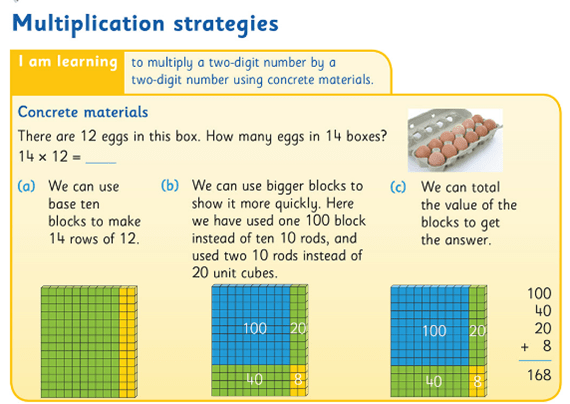Using base ten blocks to demonstrate multiplication as an area array

Similarly, in 4th to 6th classes, the approach taken should start with concrete materials (eg base-ten blocks to represent multiplication using the area model, as shown above) as demonstrated by the images in the Pupil’s books and explained in the relevant Teacher’s Resource Book (TRB). As always, the emphasis is on the children understanding the process before being introduced to the formal algorithms (abstract stage). Otherwise, they are just being taught to use a procedure to produce an answer without really understanding why or how it works.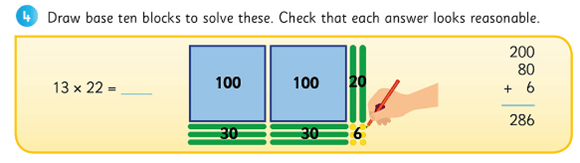Moving on from the actual blocks; drawing an area model as a pictorial representation of the calculation.

Teachers should use opportunities such as questioning, conferencing etc. to assess their children’s understanding as opposed to just checking for a correct answer.  In particular, check does the child truly understand the purpose of the zero in the second line of the long multiplication algorithm; often when questioned a child will say they need a zero or the answer will be wrong, without appreciating that they are now multiplying by a multiple of ten, so the digits must be moved up a place, hence the necessary zero as placeholder. Therefore, in the example below, 14 is first multiplied by 7, giving a product of 98 on the first line, and then 14 is multiplied by 10 (not 1) giving a product of 140 on the second line. These partial products are then added to give a final total or final product. If in doubt about a child’s understanding, return to the concrete materials and/or pictorial phase again.  Based again on the area model of multiplication, the Partial Product finder tool from the Math Learning Centre can also be very useful to demonstrate this (shown below).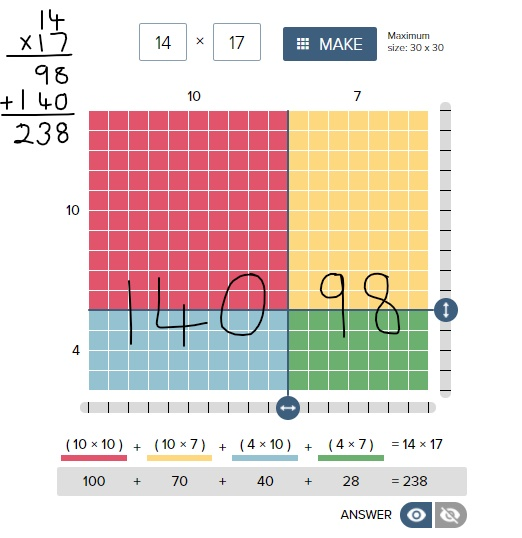If you want even more examples of the effectiveness of using the area model of multiplication, please check out this video from James Tanton. The entire facebook post is accessible here

Similarly, when considering division, while the goal is for the children to be able to divide by two-digit numbers confidently, that doesn’t necessarily have to be via the formal long division algorithm. The long division algorithm has long been a controversial element in primary mathematics. Many feel it is counter-productive to be spending so much time in 5th and 6th class learning and teaching a procedural approach to solve written calculations, when the children will have continual access to a calculator for such calculations once they hit secondary school. In fact, in many other educational systems (UK and various US states included) they have abandoned the teaching of the long division algorithm completely in preference to the ‘chunking method’ (also known as the partial quotients method). However, if the method of the long division algorithm is taught using a CPA approach, as demonstrated in the Operation Maths Pupil’s book for 5th class, it can serve to enhance children’s understanding of number and operations in general.### Language & PropertiesConsistent and accurate use of language is also an important way to emphasise the necessity of this interconnected CPA approach. The way that the number sentences for these operations are verbalised can unwittingly confuse children. If for  2 x 4, we say ‘two multiplied by four’, that implies 2 + 2 + 2 + 2, e.g. four plates with two cookies oneach. However, if we say ‘two times/groups/rows of four’, that implies 4 + 4, e.g. two plates with four cookies on each. While it is understood that the order for multiplication doesn’t matter (i.e. the commutative property of multiplication) and that the answer for both of these is the same, children don’t inherently understand this, especially at the introductory stage. And to further confuse them, when represented concretely or pictorially, both images would also look different.

Therefore, for consistency, initially teachers and students  should use  the language of ‘groups/rows of’ to describe multiplication sentences and use the word times when verbalising the multiplication symbol (×). Similarly, in division we should initially use ‘shared/divided between’ and when using the division symbol (÷) we should say ‘divided into (… equal groups)’. When comfortable with the concept of division as sharing (i.e. 12 ÷ 2 = 6, 12 divided into 2 equal groups is 6 in each group) then the children can be introduced to the concept of division as repeated subtraction (12 ÷ 2 = 6, how many groups of 2 in 12 or how many times can I take  a group of 2 away from 12).

Once the children understand that they can use the commutative property to turn around facts in order to calculate more efficiently, they should be encouraged to recognise how multiplication sentences can be distributed into smaller groups, and that smaller groups can be combined to give the same total i.e  the distributive property of multiplication. It is hoped that they will begin to recognise where these properties can be applied in order to make calculations easier. Encourage the children to read distributed number sentences using the language of ‘groups of’ or ‘rows of’, as this can aid clarity, where the numbers and symbols all together can cause confusion. The 100 dots grids on the inside back covers of Operation Maths 3 and 4 can be extremely useful for the pupils to model various arrangements/arrays, while the teacher can use the 100 square eManipulative to replicate the children’s arrangements on the IWB.Ultimately, being able to understand and apply the properties of multiplication greatly aids fact fluency. Understanding the distributive property, in particular, is key to understanding how the long multiplication algorithm works, i.e. that we multiply by the units and then multiply by the tens and then recombine these partial products. The distributive property and the ability to combine or break apart groups within factors also provides the children with strategies to mentally calculate products and quotients without having to use the standard written algorithms, for example using branching and T-charts, as featured in Operation Maths 5.

### Thinking Strategies for the Basic Number Facts

When most of us were in school, multiplication and division was largely all about tables and learning off tables. Yet, research into this area in recent years suggests strongly that memorisation and rote learning is not an effective strategy; that even when successful (which may not happen for all), the children are learning off the basic number facts, without fully understanding them, and the connections both within and between these groups of facts. Therefore, while instant recall of the basic facts (up to 10 times and divided by 10) is a primary goal of both the mathematics curriculum and of Operation Maths, Operation Maths places significant emphasis on equipping the children with thinking strategies that will enable them recall the basic facts and to compute mentally, even with numbers that are outside the traditional limits of the tables.

Some of these strategies include utilising a child’s understanding of division as the inverse of multiplication, the properties of multiplication (e.g. identity, zero, commutative and distributive) which in themselves lead into exploring connections such as doubling and halving, thirding and trebling and facts as being one set more or less another fact etc. For example, consider 9 x 5

● 9 × 5 = 5 × 9 = half 90 =45 (communtative property & 5 times as half 10 times)
● 9 × 5 = (10 x 5)- (1 x 5) = 50 – 5 = 45 (9 times as one set less than 10 times)
● 9 × 5 = treble (3 × 5) = 3 × 15 = 45 (9 times as treble, treble)
● 9 × 5 = (3 × 5) + (6 × 5) = 15 + 30 = 45 (9 times as treble plus double the treble)

That said, it would be important that these strategies are not taught purely as tricks to help arrive at an answer. Rather, the emphasis should be on the children having sufficient concrete and pictorial experiences so that they can explore and deduce the connections between groups of facts for themselves and, thus, use what they know to solve what they didn’t know.

HINT: To find out more about Thinking Strategies for Multiplication and Division, please read on here: http://operationmaths.ie/thinking-strategies-for-multiplication-and-division-number-facts/

### Mental strategies are as important as written methods

Similar to addition and subtraction, the aim is that the children will also become proficient calculating mentally, using such strategies as those outlined above, as well as using standard written methods. Once the child can use the thinking strategies to aid the recall of the basic number facts (previously referred to as tables), they can then progress to use these strategies to numbers outside of the traditional limits eg 5 x 18, 9 x 14 etc.

Again this would be very similar to the Number Talks approach to mental strategies for multiplication and division, and using the Number Talks resources accessible via the link above, alongside Operation Maths would greatly support the development of mental computation in any class.

Another important mental strategy is the ability to estimate products and quotients. Similar to the approach to Addition and subtraction, Operation Maths places particular emphasis on the development of estimation skills for multiplication and division and introduces and develops specific estimation strategies as the books progress. Once again, the emphasis should be firstly on producing quick estimates based on rounding or finding compatible numbers and secondly, on examining quickly the reasonableness of the answers e.g. number of digits. Children sometimes feel that the teacher is adding extra work by asking their students to estimate; it is important they realise that, if done quickly, estimating can greatly improve their ability to recognise errors in their own or in other’s work. However, if a child continues to have difficulties with rounding or compatible numbers, e.g. if it is taking them too much time to create an estimate, then these children should be encouraged to use front-end estimations instead, only looking at the first digit, e.g. 1,643 × 6: think 1 thousand × 6 is 6,000. While these
estimates will be less accurate than those generated by rounding, they will give the children a sense of what answer to expect in a more time-efficient way. To find out more about some of the estimation strategies, read this post.

### Problem-solving strategies

Once the children are familiar with the specific concepts of multiplication and division being taught, they should have opportunities to apply this understanding to a variety of problem solving situations, including word problems. These type of activities are typically part of the blue Work it out sections in the Pupils Books. For the children to develop their abilities to visualise the problems, they should be encouraged to use a variety of visual strategies, including bar models, branching, empty number lines, colour-coding specific operation phrases, and/or using guess and test. These various strategies are examined and developed at specific places throughout the Operation Maths books to allow the children to explore when and how they might best use them, but ultimately the child should be encouraged to use the strategy that works best for them.

In the multiplications and division chapters which are placed earlier in the school year, where the content is essentially consolidation of that covered in a previous class, some children may find the word problems quite easy to solve using a mental calculation and may be reluctant to ‘show their thinking’ in a visual/pictorial way, as they may feel that it slows them down. However, it is worth emphasising that they should get into good habits now, when the numbers involved are less challenging.

• Dear Family, your Operation Maths Guide to Multiplication & Division includes practical suggestions for supporting children, and links to a huge suite of digital resources, organised according to class level.
• Operation Maths Digital Resources: As always don’t forget to access the linked digital activities on the digital version of the Pupil’s book, available on edcolearning.ie. Tip: look at the footer on the first page of each chapter in the pupil’s book to get a synopsis of what digital resources are available/suggested to use with that particular chapter.
• For more hints and tips specific to each class level, check out the “What to look out for” section in the introduction to this topic in the Teacher’s Resource Book (TRB)
• Mental Maths handbook for Multiplication and Division from the PDST
• Number Talks book by Sherry Parrish
• Splat! Similar to Number Talks, these free resources from Steve Wyborney encourage discussion and reasoning. Play the PowerPoint presentations on your class IWB while the children use their Operation Maths MWBs to respond.
• Multiplication and Division Board on Pinterest
• Does the decimal point move? A one minute video which shows multiplying/dividing by 10/100 etc using the moving digits approach
• Can I divide by zero? A one minute video which illustrates the difficulties that arise when we consider dividing by zero
• Two more short videos from Graham Fletcher showing the progression of multiplication and division.

## Digging Deeper into … Time (all classes)

Category : Uncategorized

For practical suggestions for families, and links to useful digital resources, to support children learning about the topic of time, please check out the following post: Dear Family, your Operation Maths Guide to Time

Time is an integral element of our daily lives and therefore an essential, “need-to-know” topic in primary maths, particularly for those children with special educational needs or learning difficulties. However, it is also a very difficult mathematical concept, and one with which many children struggle, for a number of reasons:

•  Time is abstract; it cannot be touched, or manipulated, or seen (although we can see its effects when we look in the mirror!).
• The standard units of time do not reflect the familiar structures of our base-ten place value system i.e. 60 seconds = 1 minute, 60 minutes = 1 hour, 24 hours = 1 day, 7 days  = 1 week etc.
• It can be displayed in analogue and digital forms and, furthermore, digital times can use a 12 or 24 hour system.
• It is not uniform around the globe; each country belongs to a time zone and the time is different in each time zone.

Furthermore, there is a distinct difference between identifying a particular instant/moment in time (i.e. the skill of reading or telling the time) and understanding the concept of duration and the passage of time. In many cases, children may know how to tell the time, without having any real understanding of the concept of duration. Remember, that just because a child can read the digits on a digital clock/watch, or even read time from an analogue clock/watch, this does not mean they understand time as a concept.

As explained in all of the previous Digging Deeper posts, Operation Maths is based on a CPA approach. And, while we acknowledge that given the abstract nature of time is can be difficult to represent it concretely, Operation Maths does introduce the empty number line as a way to pictorially represent elapsed time (see below in post).

### Telling Time

Telling time requires the children to:

• develop an understanding of the size of the standard units of time eg days, weeks, hours, minutes
• be able to estimate and measure using units of time
• read and tell the time using both analogue and digital displays (12 hr & 24 hr).

The analogue clock has its own features that can further complicate matters; there is a “past” half and a “to” half; when reading times on the hour, the hour is said first (eg 3 o’clock) but when reading all other times the hour is said last (eg half past 3). Other valid questions that a child might have about the conventions of reading time include:

• Why do we only say half past; why not half to? (Interesting point: the German for half past three, translates literally into English as half to four)
• Why do we say half past; why not 30 past?
• Why do we say quarter past/to; why not 15 past/to?

More often than not, when teaching analogue time, teachers tend to get the children to focus on the position of the long/minute hand and to use that to tell the time eg “if the minute hand is at 12, it is o’clock”, “if the minute hand points at 6, it is half past” etc. However, this type of explanation can in itself be very confusing, with many children interpreting half past any time as 6 o’clock, quarter past any time as 3 o’clock etc.

In fact, the first thing a child should be able to read is the bigger unit of time i.e. to identify the hour (hence, the first mention of telling time in our Primary Mathematics Curriculum is telling time to the hour, in senior infants . To do this, the children should, logically, look at the hour hand, which, although it is shorter than the minute hand, can often be wider on real analogue devices, emphasizing its significance. When the hour hand points straight at a number, then it is that time; when it points half way between two numbers, it is half past the previous hour (also the lesser number). In this way, the children will be enabled to tell time in relation to the hour eg “it’s nearly 2”, “it’s just gone past 7”, “it’s around half 5”, etc. Consider also how many children, when drawing hands on a clock to show time, will often have the hour hand pointing straight at the number even if it is half past, quarter past or a quarter to the hour. Focusing initially on the hour hand rather than the minute hand, highlights the fact that the hour hand also travels around the clock as the hour passes, and doesn’t jump from one hour to the next. A real or made clock with only the hour hand can be very useful here to teach this concept. Or use the Operation Maths Clock eManipulative (pictured below), and ask the children to look only at the red hour hand. The Two Clocks problem from NRICH can also be used to reinforce the importance of the hour hand.Next, draw the children’s attention to the blue minute hand and to the blue minute markings around the edge of the clock. Logically, the minute hand has to be the longer hand because it points, beyond the numbers, at the minute markings which are furthest out from the centre, whereas the hour hand points to the hours (numbers) which are typically closer to the centre, and thus the hour hand is shorter. Emphasise that the minute hand enables us to become more accurate in our measurement of time. “O’clock” is actually an abbreviation of “of the clock”, so then when the minute hand points to the top of the clock , it is o’clock. Avoid saying “when the minute hand points to 12 it is o’clock” as the minute hand is actually pointing to the minute markings around the edge and not to the hours, which instead is the purpose of the hour hand.

At this point, it can be useful to use a cut-out paper circle (see below) to represent the clock and fold it in the centre to show both halves of the clock. Thus we can explain that when the minute hand points at the bottom/base of the clock, it is half past, as the minute hand has now passed through half of the clock. In a similar way, use the paper circle to make quarters and emphasise that when the minute hand has passed a quarter way through the hour, it is a quarter past, and when the minute hand is a quarter away from the next hour, it is quarter to. However, it should be acknowledged that, while it would be mathematically correct to say it is three quarters past the last hour (which prepares them for digital time), the convention used is to describe time in terms of how it relates to the next hour once it has passed the half-way point, at the bottom of the clock. Return to the paper circle at this point and label each half “past” and “to”. “Past” and “to” are also clearly labelled on the Operation Maths Clock eManipulative (see above), along with arrows to indicate the clockwise direction in which the hands travel.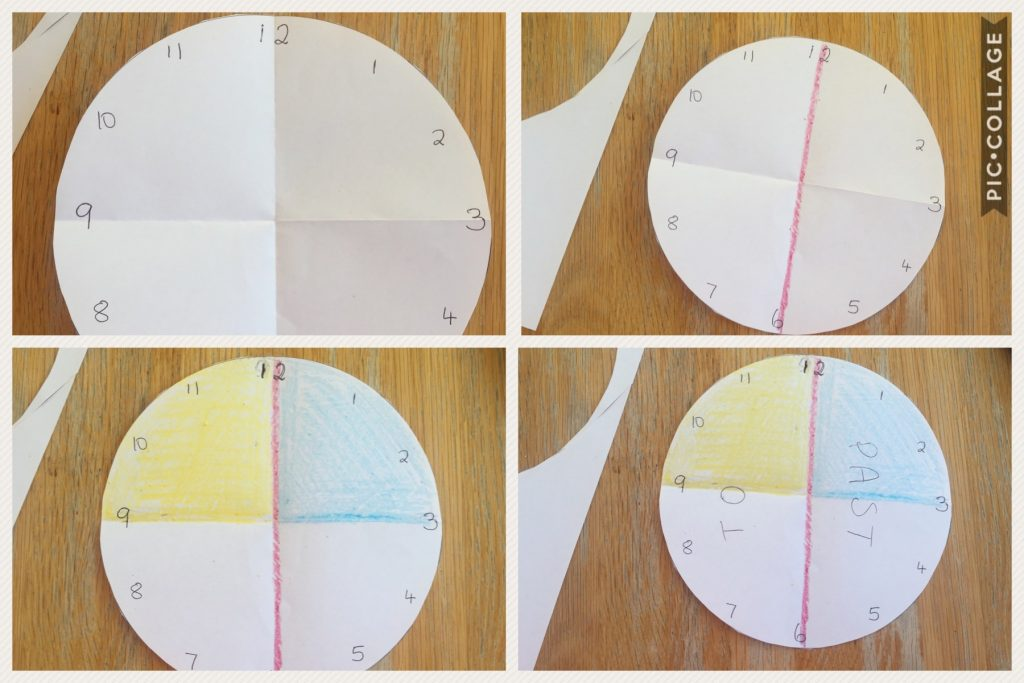When the children are ready, they should begin to measure time in minutes also (reading time in 5 minute intervals is on the curriculum for third class). Again, focus their attention on the blue minute hand and on the blue minute markings around the edge. In this way, the children may initially begin counting  the minutes in ones around the edge before realising that it is more efficient to count in groups of five, which co-incidentally, are also marked by the hour numbers. Creating and using peek-a-boo clocks can help reinforce this idea.

It is essential that when teaching digital time that the connection between analogue and digital is emphasised from the start. The Operation Maths Clock eManipulative is very useful in this regard as both clock types can be shown concurrently (see below). It is also includes the feature to hide/reveal the time in word form, as well as the feature to produce a random time on either clock, which can then be displayed manually on the other clock to match. For ease of use the teacher can also select the options that best suits the class level and ability eg hours only, time to half hours, quarter hours, five minutes, individual minutes.### Time as Duration

It is very important that the concept of time as duration is emphasised from the start. Duration of an event requires noting the starting and finishing points of time.
Developing a solid understanding of duration develops from a child’s experience  and understanding of:

• Sequencing activities (eg pictures  of familiar/daily events, seasons etc)
• The language of time such as before, after, soon, now, earlier, later, bedtime and lunchtime
• The standard cycles of time (seconds, minutes, hours, days, months, seasons etc ) which in turn follows from the sequencing of daily events.
• Measuring duration (using non-standard units initially, and then standard units)
• How long does it take to …..? Estimate & measure
• How many ….can you do in …? Estimate & measure
• What if you do it faster/quicker…? (this in turn develops an understanding of the relationship between speed and time)
• Comparing the duration of two events, (using non-standard units initially, and then standard units) eg what takes longer/shorter? How much longer/shorter is ….. than …..?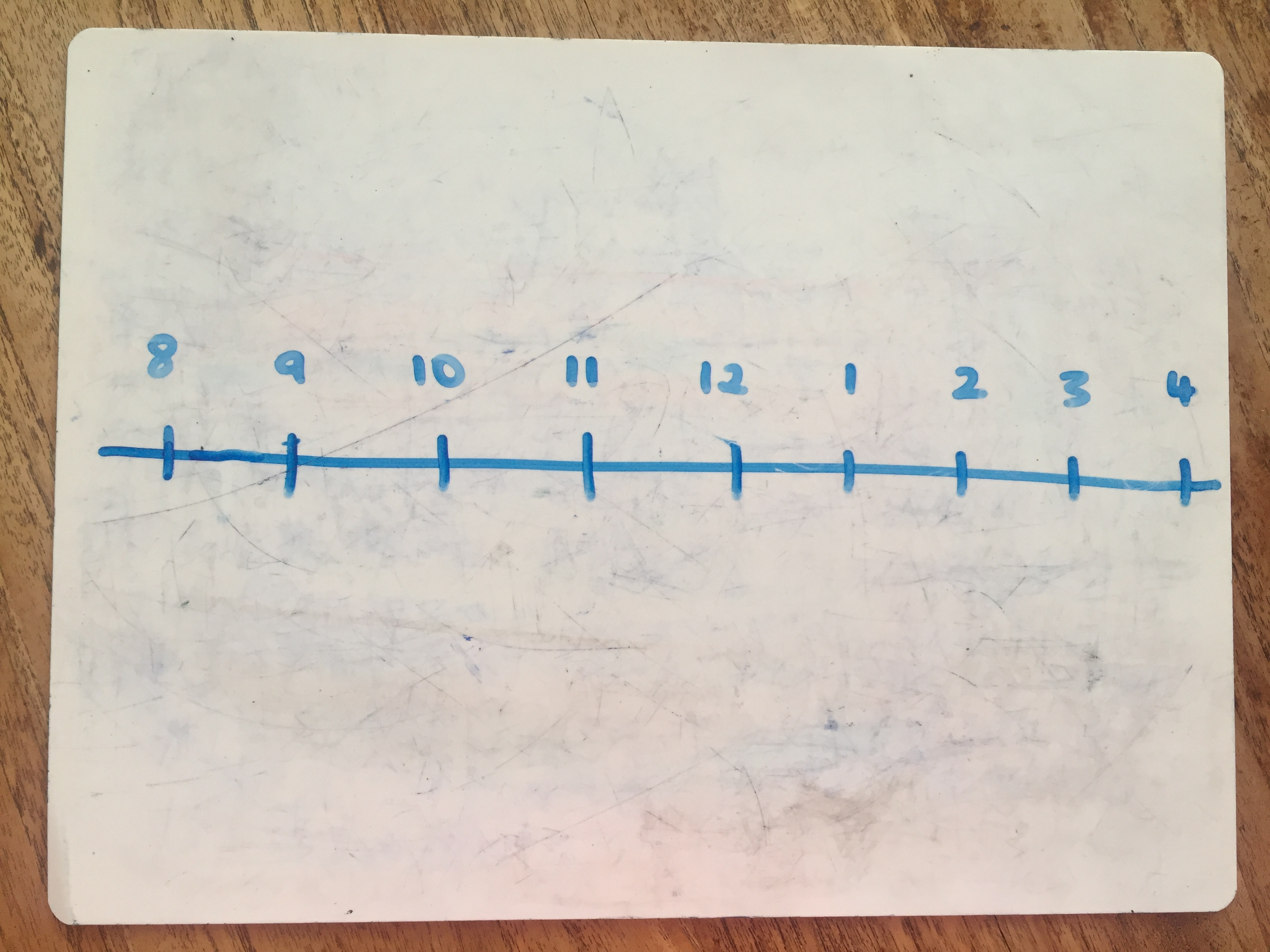Developing an understanding of duration also requires the ability to visualise the passage of time in some way. For this purpose, empty number lines, one of the key problem solving strategies used in Operation Maths, are extremely useful. This can start with drawing an empty number line on the IWB or on the Operation Maths MWBs, to which class/question appropriate details can then be added:

• What hour is after 9 o’clock? 11 o’clock? 12 o’clock?
• Ann’s school starts at 9 o’clock. What time is it 2 hours later? 2 hours earlier? 5 hours later?
• If Ann’s school finishes at 2:30 for how long is she at school?
• Umair’s school starts at 9:30 and finishes at 3:00. For how long is he at school?
• How long is it from the start of the 11 o’clock break to the start of the next break?In Operation Maths, empty number lines are presented as a viable alternative, to the traditional column method approach, for calculating time. In many other countries, the traditional column method used for calculations involving units, ten, hundreds etc., is not encouraged, or not used at all, for calculations involving hours, minutes etc. However, as our Primary Mathematics Curriculum here in Ireland, still specifies the use of subtraction to solve elapsed time problems, Operations Maths has opted to present both ways in the books.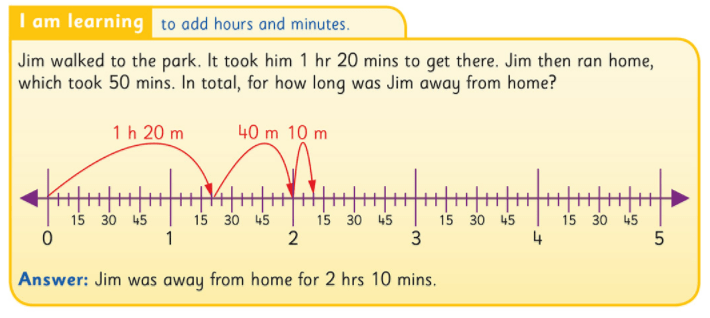To view an excellent video of students solving elapsed time problems using an empty time line, please click here.

### Other tips and suggestions for teaching time

• Refer to, and use, aspects of time as much as possible during the school day, as appropriate to the class level e.g.:
• Assign times for tasks and show interactive count-down timers on the IWB. This loop timer is particularly useful for timing stations in class.
• Reference calendar facts such as the current, previous and next day, date, month, season, etc every morning.
• Have a calendar visible in the classroom, marked with significant dates eg school play, outing, pupil birthdays etc. Ask the children to tell you how long it will be (in hours, days or weeks) until certain events (try to only use calendars that start with Monday as the first day)
• Encourage the children to wear watches themselves, with a preference for analogue, as an awareness of analogue time better develops the children ability to appreciate the passage of time.
• If buying a new clock for your classroom, try to ensure that it has the necessary features to help the children better understand how to read time.
• When writing time in digital format always use a colon (as seen on digital displays) as opposed to a dot i.e. 10:30 as opposed to 10.30. Using a dot, which is identical to a decimal point, doesn’t help the child to recognise that the system of measuring time is inherently different from our base-ten place value system.

## Digging Deeper into … Addition and Subtraction (3rd to 6th class)

Category : Uncategorized

For practical suggestions for families, and links to useful digital resources, to support children learning about the topic of addition and subtraction, please check out the following post: Dear Family, your Operation Maths Guide to Addition and Subtraction

Addition and Subtraction is always the first operation’s chapter in Operation Maths 3-6, and it is always a double chapter i.e. it is structured to be covered over 10 days/two school weeks. In Operation Maths 3-5 there is also a second Addition and Subtraction chapter (this time only a single i.e. one week chapter) in the second half of the school year to revise and re-focus on specific strategies that can be used.

### Relationship between addition and subtraction

In contrast to traditional maths schemes, which often have separate chapters for each operation, Operation Maths instead teaches addition and subtraction together, as related concepts. Teaching the operations in this way will encourage the children to begin to recognise the relationships between addition and subtraction, and indeed all the operations. Thus, the initial activities in the Discovery Book, require the children to reflect on their understanding of the concepts and to compare and contrast them.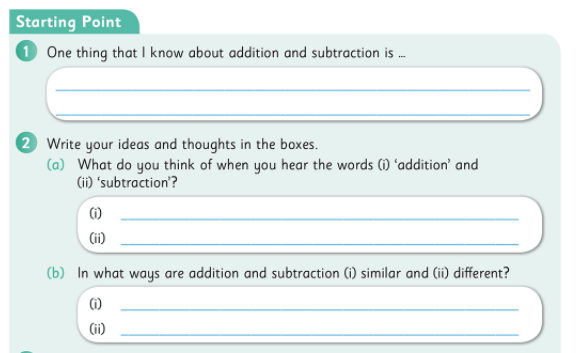In particular, the children are enabled to understand addition and subtraction as being the inverse of each other and are encouraged to use the inverse operation to check calculations.### Looking at the bigger picture

Children can often have tunnel vision (or column vision) regarding addition and subtraction calculations: they “do” the units, then the tens, then the hundreds without really looking at the whole numbers or the processes involved.One way in which you can encourage the children to look at and understand these operations better is by using a Concrete–pictorial–abstract (CPA) approach. This means the children will be moving from experiences with the familiar base ten concrete materials (e.g. straws, base ten blocks, money, the Operation Maths place value discs, pictured above) to pictorial activities (e.g. where the children draw representations of the numbers using pictures of the concrete materials or use empty number lines, bar models, etc.) and finally to abstract exercises, where the focus is primarily on numbers and/or digits.

When exchanging tens and units or tens and hundreds, reinforce that a ten is also the same as 10 units, and that a hundred is the same as 10 tens and 100 units.
The use of non-canonical arrangements of numbers (e.g. representing 245 as 2H 3T 15U or 1H 14T 5U), as mentioned in Place Value, can also be very useful to children as they develop their ability to visualise the regrouping/renaming process. The Operation Maths Place Value eManipulative, accessible on edcolearning.ie,  is an excellent way to illustrate this and explore the operations in a visual way. Worth noting also, is that the Operation Maths Place Value eManipulative and place value discs provide the only means to concretely or pictorially represent base ten materials to five whole number places (no other interactive tool is available on the internet to do this); a fact which will be of particular value to teachers of 5th and 6th classes who didn’t have a way to concretely/visually represent numbers to ten thousands prior to the inception of Operation Maths.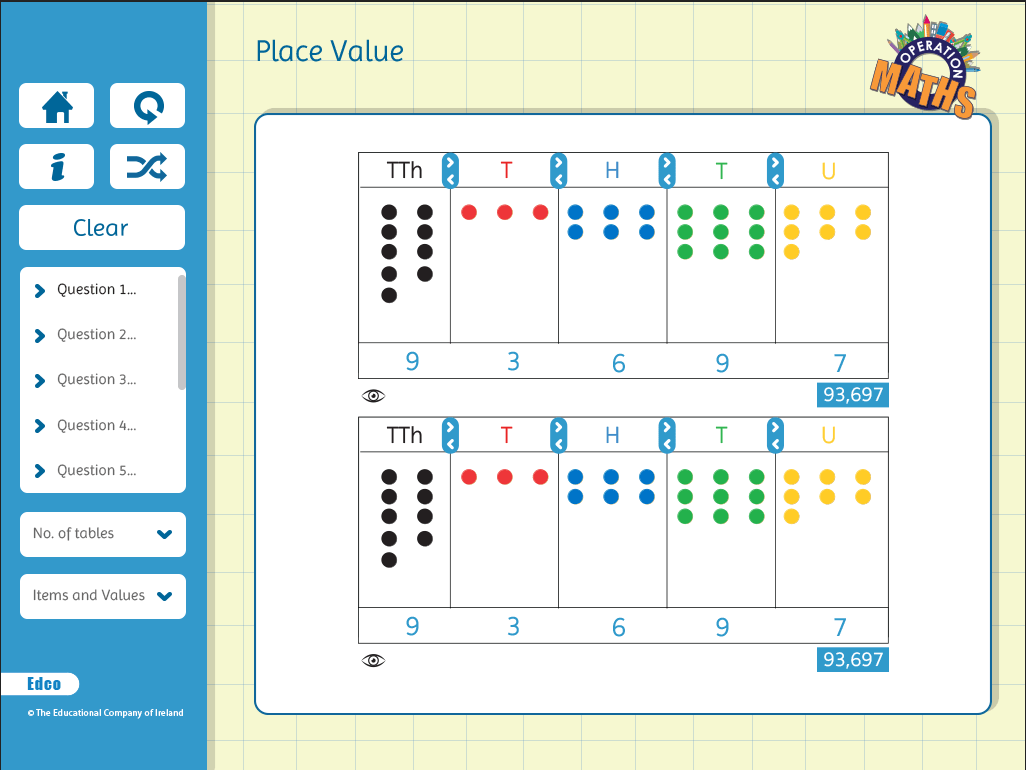### Mental strategies are as important as written methods

The traditional, written algorithms for addition and subtraction, i.e. the column methods, are important aspects of these operations. However, in real-life maths, mental calculations are often more relevant than written methods. Also, as mentioned previously, children can often have tunnel vision (or column vision) regarding addition and subtraction calculations; they ‘do’ the units, then the tens, then the hundreds, etc., without really looking at the entire numbers or the processes involved. Therefore, while the column method for addition and subtraction is a main part of this topic, equally important is the development of mental calculation skills, using such strategies as those outlined on this page from Operation Maths 6 (below)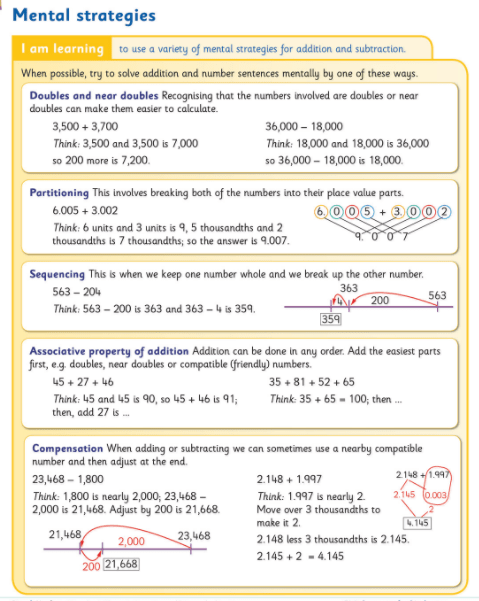Thus, one of the main purposes of the Addition and Subtraction chapters in Operation Maths is to extend the range of mental calculation strategies the children have and to enable them to apply the strategies to numbers of greater complexity i.e. for the children to become efficient and flexible, as well as accurate. As the same calculation can often be done mentally in many different ways, the children have to develop their decision-making skills so as to be in a position to decide what is the most efficient strategy to use in each situation.

It is worth noting that the page from Operation Maths 6 pictured above serves as a synopsis to remind the children of all the strategies they explored individually in the previous Operation Maths books. That said, if the sixth class children are new to Operation Maths and have never encountered these strategies before, they may need to progress at a much slower pace than those who have been using the programme previously, or who may have encountered these strategies, for example a class who used Number Talks. As mentioned in a previous post, the Operation Maths mental strategies listed below are very similar to, and in some cases identical to, those used in Number Talks (if different terminology from Operation Maths is used in Number Talks, the Number Talks terminology is given in brackets).

• Doubles and near doubles
• Number bonds of 10, 100 and 1,000 (Making tens)
• Friendly or Compatible numbers (benchmark/friendly numbers)
• Partitioning (breaking each number into its place value parts)
• Compensation
• Subtraction as take-away (removal/deducation)
• Constant difference subtraction (see below)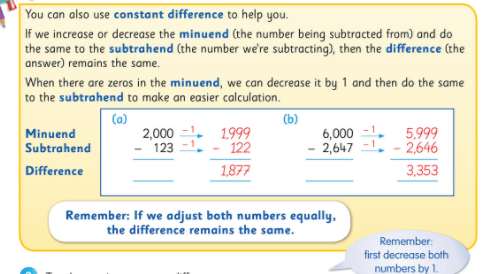Operation Maths also places particular emphasis on the development of estimation skills for number and introduces and develops specific estimation strategies as the books progress. Again, the emphasis is on the children contrasting and comparing these strategies and choosing the most efficient strategy each time. To find out more about some of the estimation strategies, read this post.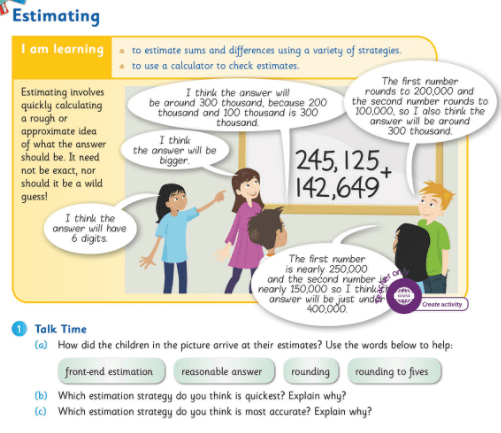Therefore, ask the children, as often as possible when meeting new calculations, can they do it mentally, and how, so that they become increasingly aware of a range of mental calculation skills and approaches. In this way the children will also be developing their decision-making skills, so as to be in a position to decide the most efficient strategy/approach to use.

### Problem-solving strategies

One of the main aims of Operation Maths 3-6 was to introduce both teachers and pupils to a logical problem solving approach (i.e. RUCSAC) , complemented by specific visual problem solving strategies which develop in complexity as the child progresses through the senior classes.

A key step in the RUCSAC problem-solving approach is the ability to read a word problem meaningfully, and highlight the specific operational language or vocabulary. This is reinforced with activities in the Discovery Book (see below) where the children colour-code the specific phrases and then transfer them to their Operations Vocabulary page towards the end of their Discovery Book for future reference.You will notice that the problems have no numbers to distract the children, so that they can just focus on the language of the problems and the operations that may be inferred by the context of the story. These type of “numberless word problems” are being used more and more by practitioners in order to deepen children’s understanding of the concepts involved.

Another key step in the RUCSAC approach is the ability to create to show what you know, where the child makes a representation of the word problem in another form. Bar models are ideal for use with operational word problems. Introduced initially in Operation Maths 3, the use of bar models is developed through Operation Maths 3-6 to include bar models suited to other types of word problems.

Empty number lines can also be used to represent addition and subtraction problems (see below). In the senior books, the children will use both strategies to represent word problems and compare and contrast the two strategies. Ultimately, it is hoped that the children will use the strategy that they are most comfortable with. For more information on problem-solving strategies please consult the guide to problem-solving strategies across the scheme in the introduction to your Teachers Resource Book (TRB) or read on here.### Communicating and expressing thinking

Being able to explain your mathematical thinking is a very powerful tool, and one that can greatly aid the learning and understanding of both the speaker and the listener(s). Encourage the children to verbalise how they did their calculations (mental or written) to provide you with a window on their thinking. When talking about decimal numbers, encourage children to use fractional language as opposed to decimal language, i.e. ‘6 hundredths plus 4 hundredths is ten hundredths’ etc.Another way to communicate and express thinking is via jottings. These are informal diagrams that both show and support thinking, and when used as a part-mental approach, serve as an intermediate stage between concrete materials and the abstract calculation. Their use should be encouraged as much as possible (e.g. “use jottings to show me your thinking”) until the child is confident enough to do the whole calculation mentally or using a traditional written form. The main jottings used in Operation Maths are empty number lines (pictured above) and branching (pictured below) to show part–whole relationships and/or explore compensation.• Dear Family, your Operation Maths Guide to Addition & Subtraction includes practical suggestions for supporting children, and links to a huge suite of digital resources, organised according to class level.
• Operation Maths Digital Resources: As always don’t forget to access the linked digital activities on the digital version of the Pupil’s book, available on edcolearning.ie. Tip: look at the footer on the first page of each chapter in the pupil’s book to get a synopsis of what digital resources are available/suggested to use with that particular chapter.
• Mental Maths handbook for Addition and Subtraction from the PDST
• Number Talks book by Sherry Parrish
• Addition & Subtraction Board on Pinterest
• This short video from Graham Fletcher showing the progression of addition and subtraction from the infant classes to the formal written algorithm, with three and four-digit numbers, is also worthwhile viewing:

## Digging Deeper into … 2D Shapes (3rd – 6th)

Category : Uncategorized

For practical suggestions for families, and helpful links to digital resources, to support children learning about the topic of 2-D shapes, please check out the following post: Dear Family, your Operation Maths Guide to 2-D Shapes

### Overview of 2D-Shapes:

The following are the new 2D shapes, to which the children are formally introduced, in the senior end of primary school:

• 3rd class: hexagon
• 4th class:  Equilateral, isosceles and scalene triangles; rhombus & parallelogram; pentagon and octagon
• 5th class: Polygons, quadrilaterals, trapezium
• 6th class: Kite*

* while the kite is not specified on the Primary Mathematics Curriculum (1999) for sixth class, it has been included in Operation Maths 6 as it features on the curricula for 5th/6th grade in many other countries.

As with every topic in Operation Maths, a CPA approach is also recommended for 2D shapes:

Concrete: Using concrete geometric shapes for classifying and sorting; identifying examples of 2D shapes and tessellations in the environment.
Pictorial: Tracing around shape templates to make reproductions that can be manipulated, folded, partitioned and combined; using lollipop sticks, geostrips or geoboards to create representations of 2D shapes; using the Operation Maths Scratch lessons accessible on edcolearning.ie to draw various shapes.
Abstract: Answering shape questions with no visual references/supports; suggesting the number of lines of symmetry on a shape without folding or drawing to explore the same; identifying the resulting shape when a given shape is rotated, flipped etc.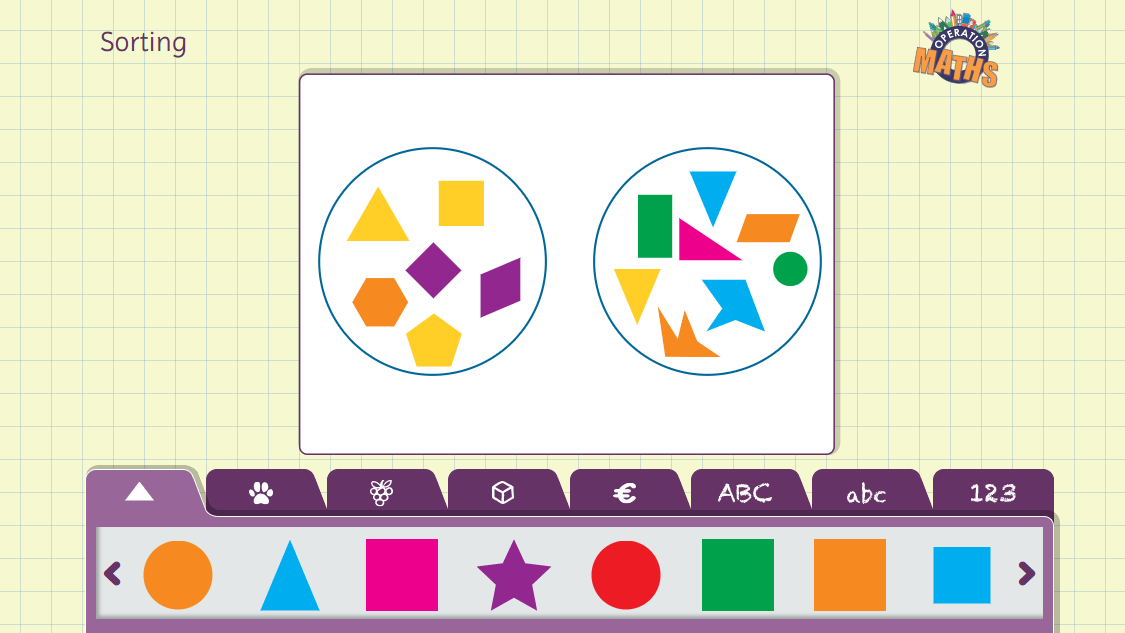### Properties of 2D Shapes:

Don’t let the list above, of 2D shapes by class, fool you; it shouldn’t create an incorrect impression that the primary focus is on identifying shapes, or that we should look at one type of 2D shape exclusive of others. Rather, the focus should be on the children examining the properties of each 2-D shape, describing it according to these properties and contrasting it with, and comparing it to, the properties of other shapes, rather than on just naming the shape. For example, what makes a square a square? How is a square similar to, or different from, a rectangle? Could an argument be made to say a square is also a rectangle? Could an argument be made to say a rectangle is also a square?

Therefore, any new 2D shapes that the children encounter should be compared to the 2D shapes with which they are already familiar. And, as the 2D shapes chapter in Operation Maths always follows on from the topic of Lines and Angles, when exploring properties, reference should also be made to the number and type of angles within the shape, the number and types of sides (parallel, perpendicular etc) and whether the shape is regular or irregular.

### Common misconceptions:

Categorising 2D shapes  separately: As mentioned previously, children often don’t recognise a square as a type of rectangle, a rectangle as a type of parallelogram, a rhombus as a type of parallelogram etc. This can often be the case if the children are focused primarily on naming the shape and then compartmentalising it in a category, as opposed to examining its properties and exploring how it may have proprieties in common with other shapes.

It can be useful here to display 2D shapes to the class using a subgroup structure (like this one here) so that the children can appreciate how, for example, a square is also a rectangle, is also a parallelogram, is also a quadrilateral, is also a polygon.

Constancy of shapes: Many children don’t recognise that a  shape remains constant, irrespective of its placement in space. In particular, a square sitting on its vertex is often incorrectly labelled as a diamond. The children should be encouraged to draw or trace around shapes on their MWBs and then rotate the shape in order to appreciate its constancy.

Regularity: Children may not recognise a five-sided figure with sides of same length as being a regular shape. It is as if the terminology “regular” implies to them that the shape should be common-place i.e. regularly occurring. For this same reason, a child will often say a rectangle and a circle are regular shapes, given their familiarity with these shapes from the junior classes, even though they are officially classified as irregular shapes. Challenging this misconception will require plenty of sorting activities where shapes are classified as regular or irregular (see Ready to Go activities below).

When creating a class display of shapes eg rectangles, triangles, etc., instead of using just one qualifying shape to illustrate the term, use many and use varied ones. Enlist the help of the class: “I want to make a display of triangles/parallelograms but I want the triangles/parallelograms to all be different. Can you draw and cut some out for me?” Such an activity would quickly reveal those who appreciate the required properties for each shape and those who don’t. Remember to also position the shapes in various ways so as to reinforce that the shape remains constant, irrespective of placement.

Identifying 3D objects as 2D shapes: This is a very problematic area. It often happens that when asked to find a circle in the environment, a child suggests a ball (a sphere) or a cube might be suggested as a square. When asking to identify 2D shapes in the classroom or at home, we must be careful how we respond to the answers so as not to reinforce these misconceptions. For example, if a child suggests that the door is a rectangle, when it is in fact a cuboid, emphasise that a part of the door is rectangular eg

• Which part of the door is like a rectangle?
• Are there any other parts of the door that are like a rectangle?
• Can you see any other rectangles on the outside of the door? How many?
• Are they all the same or different?

Asking the children to draw around solid 3D objects in order to produce flat 2D shapes can also be useful here.

### Coordinates (6th class)

The concept of plotting and reading coordinates is introduced in 6th Class. There are plenty of examples of coordinates in the children’s environment, e.g. map reading, car parks and board games such as chess and Battleship. Allow the children to practise reading coordinates on maps and on board games, then progress to using two digit coordinates in maths. Make sure they first read the horizontal coordinate and then the vertical coordinate.### Operation Maths Digital Resources:

Don’t forget to access the linked digital activities on the digital version of the Pupil’s book, available on edcolearning.ie . These include:

Ready to go Activities: based on the Sorting eManipulative, these enable the various shapes to be sorted according to class-appropriate criteria, or enable tessellating patterns to be made. The Ready to go activities all have suggested questions inbuilt on the left-hand side of the screen that the teacher can just click to reveal and hide. Remember, when sorting, the focus should be on the properties of the shapes not their names; that said, you can also ask the children to identify the shapes, if known, as an extra dimension to the activity.Create activities: (all classes) again using the Sorting eManipulative, these are less structured that their Ready to go counterparts. Instead, the teacher should click on the yellow “Create new example” button on the bottom of the screen, and then use the sorting eManipulative to explore the shapes as they see fit. The teacher can use a previous Ready to go activity to inspire the create activity or come up with a completely different activity of their own using the almost limitless possibilities of the sorting eManipulative.

Write-Hide-Show videos: These explore tessellations (3rd class) and different types of triangles (5th class). They encourage the children to look and respond to the questions by answering orally or on their MWBs.

Maths Around Us video (6th class): which examines different types of triangles from the environment.Scratch-based programming lessons with instructions on how to draw 2D shapes  (3rd class) and hexagons (3rd, 4th, 5th classes), scalene triangle, pentagon and octagon (4th class), different types of triangles (5th class) and plot 2D shapes on a grid (6th class).## Digging Deeper into … Representing and Interpreting Data (3rd-6th)

Category : Uncategorized

For practical suggestions for families, and links to useful digital resources, to support children learning about the topic of data, please check out the following post: Dear Family, your Operation Maths Guide to Data

### Data Analysis Process

Data analysis, whether at lower primary, upper primary, or even at a more specialised level of statistics, is essentially the same process:

• It starts with a question, that doesn’t have an obvious and/or immediate answer. Information is then collected relevant to the question.
• This collected information or data is represented in a structured way that makes it easier to read.
• This represented data is then examined and compared (interpreted) in such a way as to be able to make statements about what it reveals and, in turn, to possibly answer the initial question (if the question remains unanswered, it may be necessary to re-start the process again, perhaps using different methods).

• What is the most common eye/hair colour in our class?
• Which fruit/pet do we prefer?
• How did we come to school today?
• What candidate would we vote for?
• What is the temperature each day?
• How many children are absent from school every day?

When choosing a question it is worth appreciating that some questions might not lend themselves to rich answers. Take, for example, the first question above; once the data is collected, and represented, there is not that much scope for interpretation of results other than identifying the most common eye/hair colour and comparing the number of children with one colour as being more/less than another colour. However, other questions might lead to richer answers, with more possibilities to collect further information, to make predictions and to create connections with learning in other areas. Take, for example, the question above about travel; the children could be asked to suggest reasons for the results e.g. can they suggest why they think most children walked/came by car on the day in question, whether weather/season/distance from school was a factor and to suggest how the results might be different on another day/time of year. Thus, the children are beginning to appreciate that data analysis has a purpose i.e. to collect, represent and interpret information, so as to answer a question. And, in the case of the questions about temperature and number of absences, the children may begin to appreciate that it is too much to give the specific details for each individual day and that a figure to represent a larger set of numbers (eg the average) is preferable in some situations.

### Content overview

A quick glance at the curriculum content for representing and interpreting data for these classes, reveals the following:
3rd class: pictograms, block graphs, bar charts
4th class: pictograms, block graphs, bar charts and bar-line graphs incorporating the scales 1:2, 1:5, 1:10, and 1:100
5th class: pictograms, single and multiple bar charts and simple pie charts; calculating averages
6th class: pie charts and trend graphs; calculating averages

In Operation Maths for 3rd and 4th classes, representing and interpreting data is specifically taught in September, at the beginning of the school year, so that the children are enabled to incorporate these skills into other subject areas where possible e.g. reading and interpreting tables and graphs, collecting and displaying data in science, geography etc.

In 5th and 6th classes, representing and interpreting data is taught later in the school year, after the children have encountered degrees in lines and angles and the circle in 2D shapes, as this content is necessary prerequisite knowledge for creating pie charts. In these classes, representing and interpreting data is also taught as a double chapter (two week block), to allow for the extra time required to explore averages.

### CPA

As with every topic in Operation Maths, a CPA approach is also recommended for representing and interpreting data: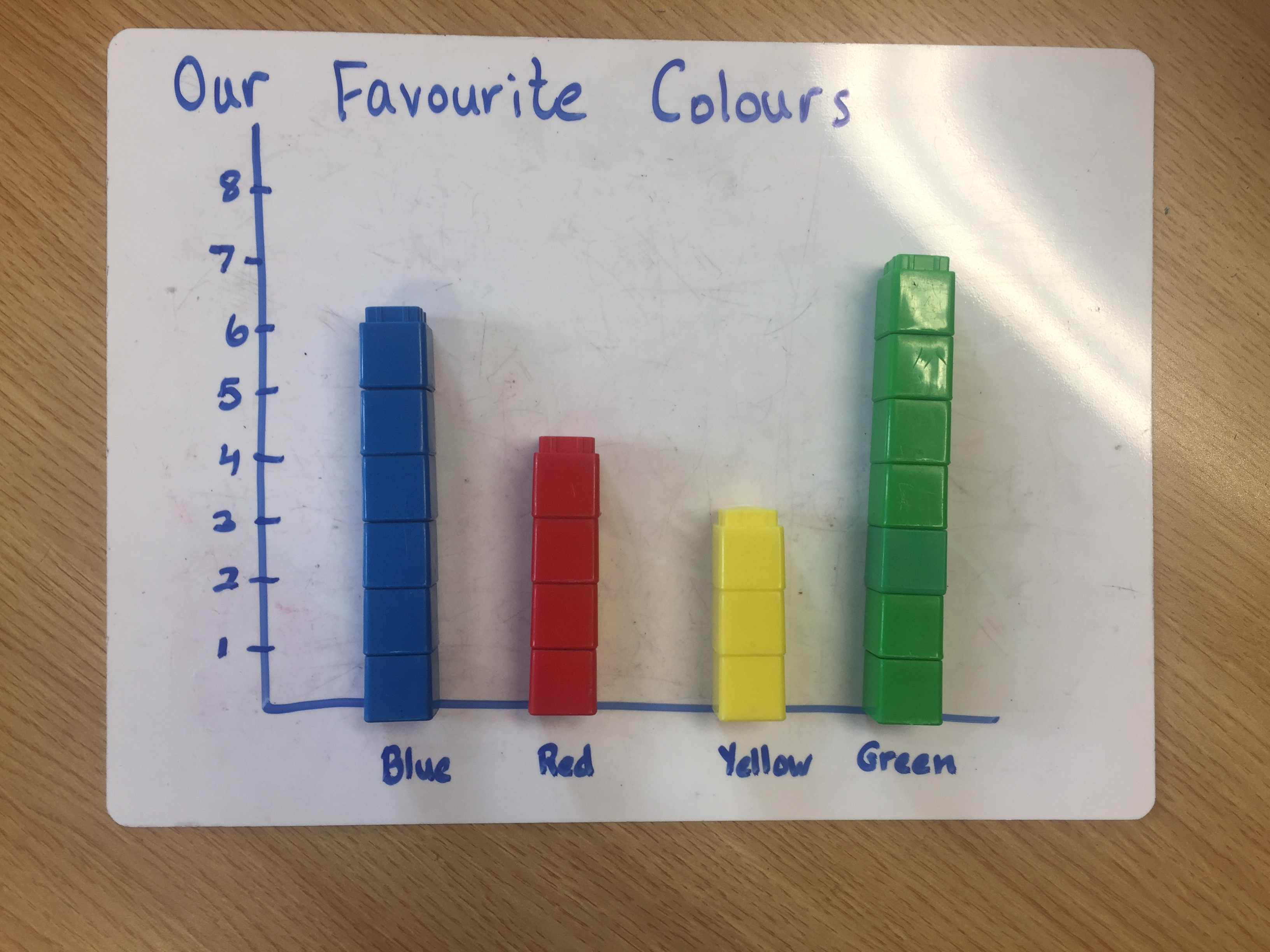Concrete: Using real objects to sort and classify eg the number of different colour crayons in a box, the different type of PE equipment in the hall etc; using unifix cubes, blocks, cuisinere rods etc to represent data; using cubes to introduce and explore the calculation of averages.
Pictorial: using multiple copies of identical images to make pictograms; using identical cut out squares/rectangles to make block graphs etc, using folded circles to make pie charts, using bar models to calculate averages.Abstract: the final stage, where the focus is primarily on numbers and/or digits eg reading and interpreting the scale on a graph where all the scale intervals are not given; calculating averages without pictorial or concrete supports.

### Interpreting data

For children to become comfortable interpreting tables and graphs it is vital that they have plenty of opportunities to look at and read a variety of tables and graphs. This shouldn’t be limited to just the tables and graphs in their maths books. In particular, data sets that are relevant to them, such as soccer league tables can be a great way to encourage the children to appreciate how relevant this strand units is to them.

Utilize every opportunity to expose them to real-life examples of data from print and digital media and use purposeful questions to highlight the features of the graph:

• What is the title of this graph/chart?
• How is the information displayed? Horizontally or vertically?
• What type of chart/graph was used?
• Why do you think this graph type was chosen? What other types would have been suitable?
• What key information is required to interpret the data (eg scale intervals, labels on the axes, a key for piecharts)?
• Is there information missing that would have been useful to get a better insight into the data?

The children can be asked to create questions based on the graph/chart and swap with a partner to answer. When they become adept at producing charts themselves (see next section) they can also be asked to represent the data using a different chart type.

One of the most common mistakes that children make when interpreting graphs is misreading the scale. Always draw children’s attention to this first, and ask them to identify the scale interval and what it means for the bars/blocks/points etc on the graph. The graph quiz on That Quiz provides lots of extra practice for this skill. The quizzes are also very customisable, with options to show pictograms, bar charts, trend graphs (line) and pie charts (circle), easier or normal content, and a variety of question types. Another similar activity is this one from MathsFrame which offers three different levels of questions on bar charts.

A very interesting  and very different way to explore interpreting data is to show the children graphs where much of the key information is missing initially, but is then slowly revealed as the children share their thoughts and ideas. Following on from Brian Bushart’s work on numberless word problems, many teachers have used graphs to create “slow reveal” activities or “notice and wonder graphs”, and have very generously shared these online for other teachers to use. Some of these include:

### Representing dataAs mentioned previously, where suitable children should begin to represent data themselves using concrete materials. They can build block graphs using cubes or blocks, laid flat on a piece of paper or their Operation Maths MWBs. These should all start from the same baseline and the children should also write in labels for the axes and a title.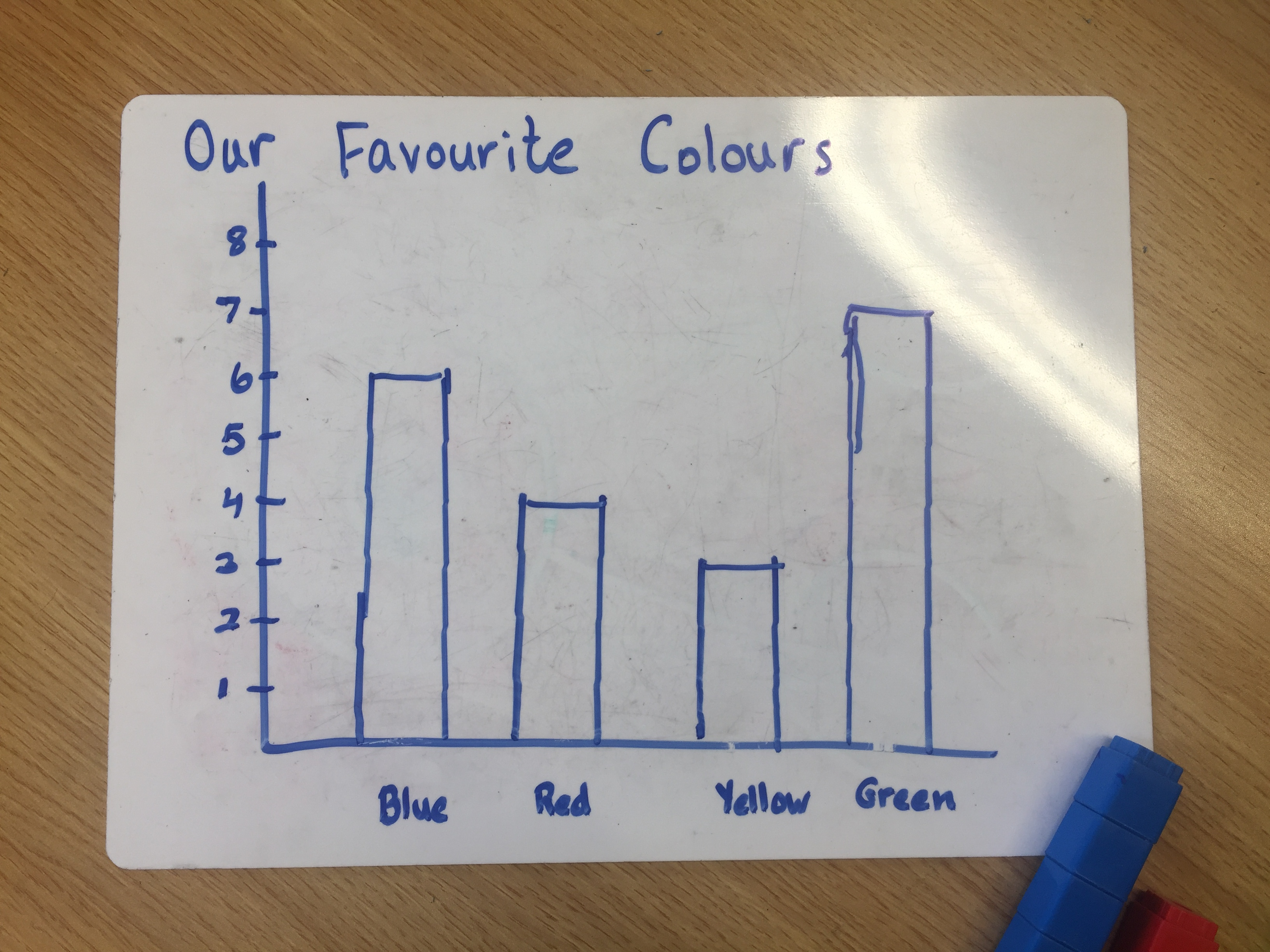As a development, they can then trace around the stacks of cubes and remove the cubes to have a pictorial representation of the concrete. Using cubes like this to represent 1:1 quantities can in turn lead children to see a need for one cube to represent more than one, ie scales of 1:10, 1:5 etc, especially if there are not enough cubes to represent the data or there is not enough space.

The next step could be to have small squares or rectangles of identical pieces of paper which can then be pasted onto a page to display the information. This can work particularly well for pie charts; cut out a circle of paper and divide it by folding into eighths; the circle can be left whole and the folds outlined in pencil/marker or the eighths can be cut up. A groups of eight children can then use either of these to show data like their favourite ice-cream flavour or TV programme. In this case, because the amount of data gathered is limited, the choices/categories should be limited, also, to three or four.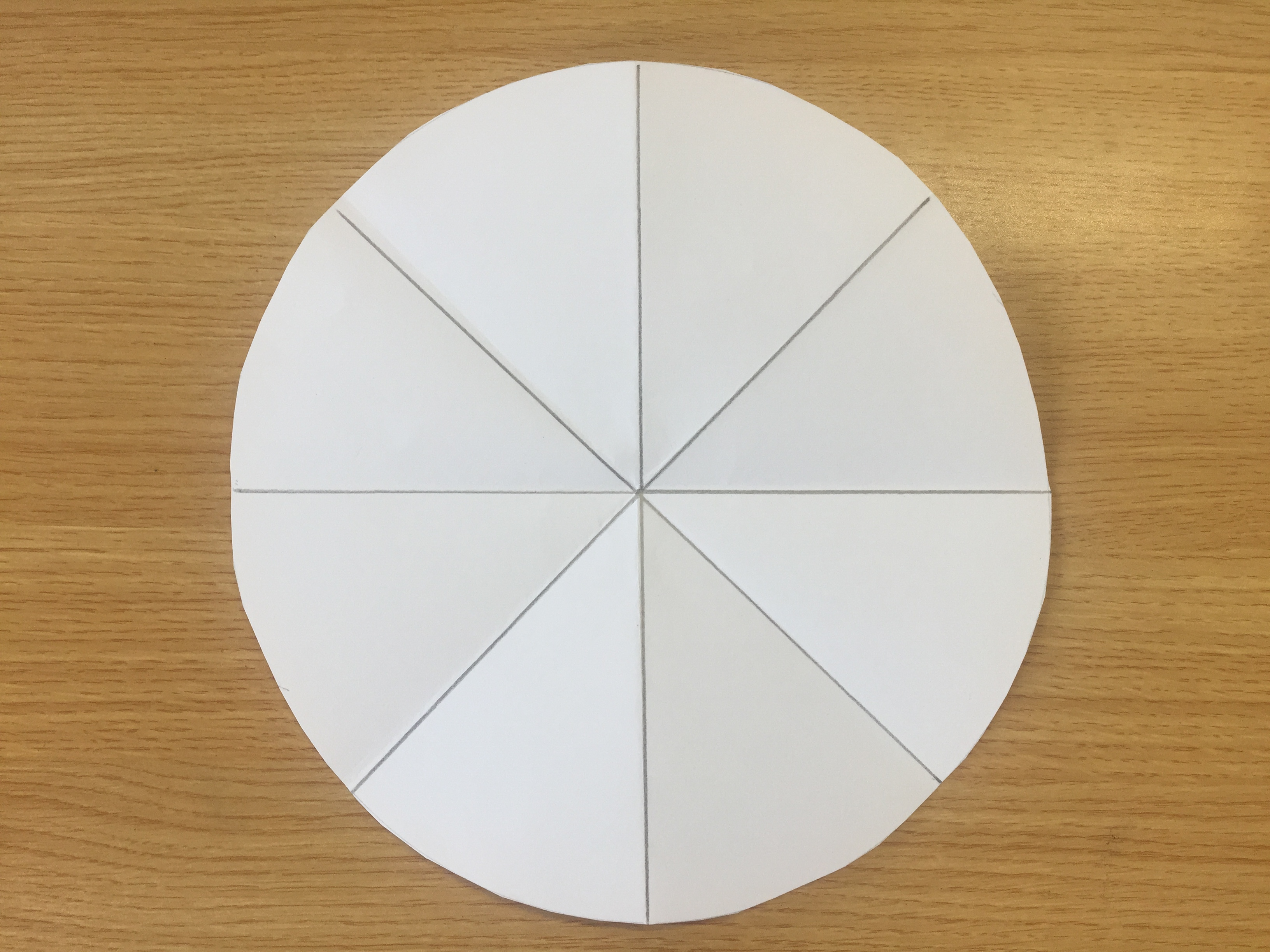If the children are also collecting the data to make a graph or chart, they will need to come up with a system to accurately collect and record this data. This will usually involve compiling a type of table with three columns; the first column to list the categories, the second to record tally marks and the third to total the tally marks. When introducing tally systems the children could use lollipop sticks to explore and make tally marks.

For children, drawing their own graphs can present many difficulties. Some common mistakes that can be made include:

• Incorrectly transferring the data from the table to the graph.
• Omitting the graph title and/or category titles on the axes.
• Using an inappropriate scale for a specific graph.
• Not setting the scale at regular, even intervals
• Zero being incorrectly located somewhere other than at the base line/axis.

And in other cases, it can just be a lack of neatness and exactness that reduces the quality, and readability, of a hand-draw graph. To overcome the difficulties associated with hand-draw graphs, the children could use either an online or offline computer application, all of which can produce very impressive results. Listed below are a small sample of those available; click on any of the links to access a tutorial or the application itself.

### Calculating averages

Averages are introduced for the first time in 5th class and the children should have ample opportunities to explore this concept concretely and pictorially, before being given the formula to calculate the average of a set of numbers. Initially, the concept should be introduced as sharing amounts out to be fair/balanced: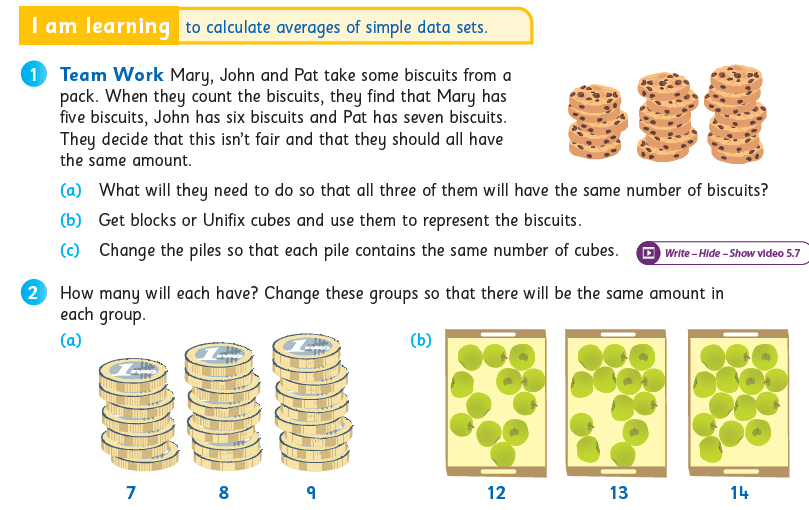Through plenty of concrete and pictorial opportunities to balance these separate quantities, it is hoped that the children begin to see a connection between the total number of items and the balanced quantity or average: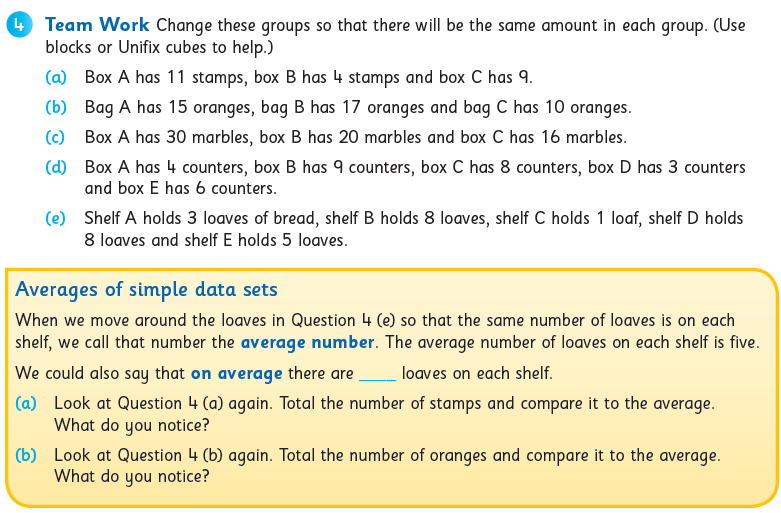Bar models, one of the key problem-solving strategies used in Operation Maths, are very useful here, where comparison models can be used to compare the total of the averaged quantities with the total of the individual quantities. They are also used in Operation Maths 6 to calculate the extra number(s) when the average increases or decreases, a concept which can be very difficult to reason if no pictorial structures are used to help visualise the relationships.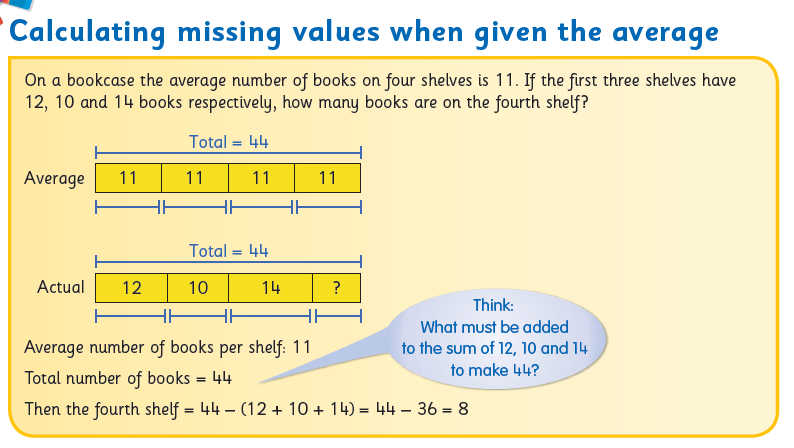You can also check out this video to see how bar models can be used to solve averages:

## Digging Deeper into … Lines and Angles

Category : Uncategorized

For practical suggestions for families, and links to useful digital resources, to support children learning about the topic of place value, please check out the following post: Dear Family, your Operation Maths Guide to Lines and Angles

### Overview:

As can be seen from the overview table below, this topic is initially introduced to the children in 2nd class via “turns” and “square corners” and then develops with increasing complexity in 3rd- 6th classes.

 2nd 3rd 4th 5th 6th Lines vertical horizontal parallel perpendicular oblique Angles Full, half, quarter turns, square corner Right angle Greater/less than a right angle Acute angle Obtuse angle Straight angle Reflex angle Measuring and constructing in degrees Sum of angles in triangle  = 180° Sum of angles in quadrilateral  = 360°

As with every topic in Operation Maths, a CPA approach is also recommended for lines and angles:

• Concrete: allow sufficient time for the children to explore making turns, lines and angles with suitable concrete materials (e.g. the children themselves, lollipop sticks, straws, geo strips, construction materials, real-life examples from the school and home environment)
• Pictorial: activities where the focus is on drawing angles or lines on paper, MWBs etc
• Abstract: the final stage, where the focus is primarily on numbers,  digits  and or letters to represent variables eg given the measure of two angles in a triangle calculate the third angle.

### Lines:

Through exploration and activities, it is important that the children realise that:

• A line can be classified and identified according to its position and its relationship to another line.
• A single line can be horizontal, vertical or oblique but a single line cannot on its own be parallel or perpendicular; there must be two or more lines.
• Parallel lines do not all have to be the same length to be parallel.
• Parallel lines do not have to be horizontal or vertical, they can also be oblique.
• Perpendicular lines do not have to have a horizontal and vertical line, (again they can be oblique) but there must be at least one right angle where the two lines meet.

Lines can be drawn on the Operation Maths MWBs and then rotated to reinforce this point.
It is also worth noting that in maths, when we use the word “line”, it should be assumed that this is always straight; only if the word curved is given should it be assumed otherwise.

### Angles

In order for the children to recognise angles in terms of rotation, it is preferable initially, for the children to investigate the angles in their environment that are dynamic, (where the angle can be easily made bigger or smaller by increasing or decreasing the distance between the two lines) e.g. a door opening and closing, a scissors cutting paper, the angles made by the hands of a clock. The children can then proceed to examine static angles (where the angle is fixed) e.g. in 2-D shapes or 3D objects.Operation Maths, Pupils’ Book 3: on the digital book, click on the icon on the bottom right to access a “Ready to go” activity.

In second class (and revised at the start of third class), the concept of rotation of an angle is taught through the terms quarter-turn, half-turn and full turn. Ideally, this should be introduced concretely by getting the children themselves to do half-turns and quarter-turns, and to turn in clockwise and anticlockwise directions:

• In the classroom, the children start facing the board/front of room and make half/full/quarter turns to left/right as directed by the teacher.
• Repeat, but this time with different starting points
• Repeat, but this time after the teacher gives the directions the children must say where they will be facing, before they do the actual turn. The children could also record their predictions quickly on their Operation Maths MWBs

The children will be also be asked to identify 90º angles as square corners (2nd class) or right angles (3rd class). This will also be reinforced as part of the 2D shapes chapter. The children can be asked how they might decide if a corner/vertex of a shape is a square corner/right angle. Prior to the introduction of the protractor, something as simple as a corner torn from a piece of paper would suffice as an instrument with which to measure these angles.

The children should be enabled to classify angles according to the criteria appropriate to their class level (see table above). In particular, the ability to identify angles as acute (or less than a right angle), obtuse (or greater than a right angle) or reflex will greatly help the children, to later, estimate the measure of the angle in degrees, and to accurately measure and construct angles when they encounter this in 5th and 6th classes.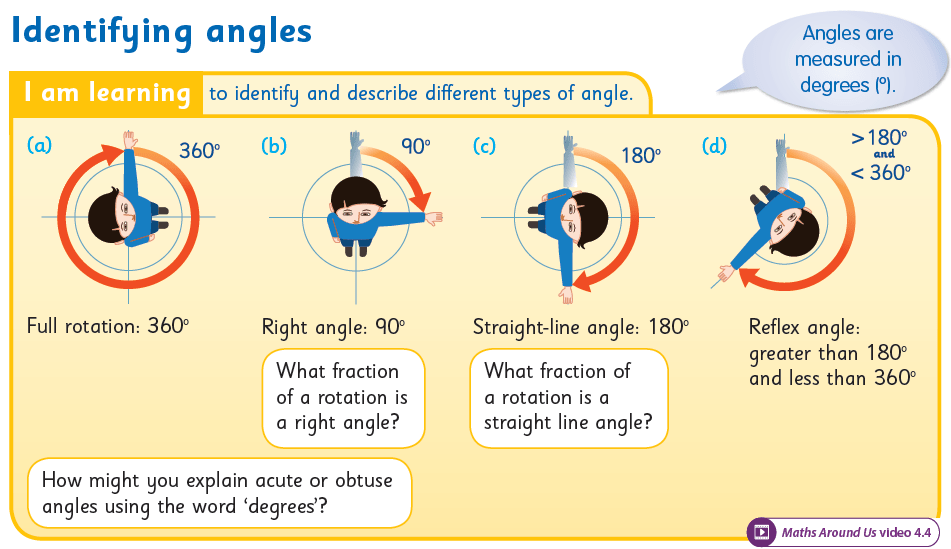Operation Maths, Pupils’ Book 5

It is important to constantly reinforce the children’s understanding of what an angle actually is, i.e. an amount of turn and that this can be represented by two adjoining lines, one showing the starting position, the other showing the point after the turn. Return to concrete examples if necessary; the children stretch out two arms in front and, leaving one arm in original position, they move other arm a certain amount (90 º, 180º etc). This could also be repeated using geostrips, connected at one end using a brass clip, so as to be able to move one of the ‘arms’. Such concrete experiences also link well to measuring using a protractor; the original arm is the ‘base’ line.

### Measuring and constructing angles (5th & 6th classes)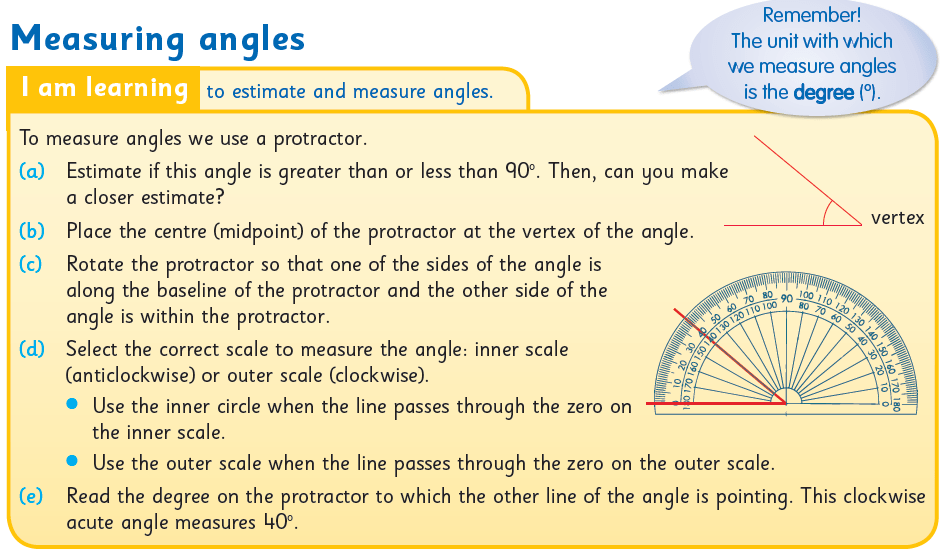Operation Maths, Pupils’ Book 5

Using degrees to describe angles is introduced in 5th class, which develops to include measuring and constructing angles using degrees. This necessitates the use of a protractor for the first time, which in itself can lead to difficulties. The child may be unsure where exactly to place the protractor; this can come from a lack of understanding of what an angle actually is and where the angle actually is. Also, a child can be uncertain of which scale to use to measure the size of the angle eg for an acute angle measuring 45º, the child writes down 135º.

To reduce the likelihood of this arising you can ask some/all of the following questions:

• What important tips would you give to a person about using a protractor?
• How do you know which scale to use on the protractor?
• What type of angle is this? How do you know? (To save time, they can write A, O or Re for Acute, Obtuse or Reflex).
• Estimate the measure of the angle to the nearest 10º. Is your estimate/measurement sensible? Why?
• How can you use what you know about acute and obtuse angles to check your measurement?

You can also watch some of the tutorial videos for using a protractor on the internet, such as the one below, for example. These videos can be a very visual way of demonstrating this skill. See also the list of digital resources for 5th and 6th classes on the following post: Dear Family, your Operation Maths Guide to Lines and Angles.

Angles is another area where it is important for the child to check the reasonableness of the answer. First, the child needs to identify whether the angle is acute or obtuse. Then, if measuring an acute angle, the measurement must be less than 90º. If it isn’t, then the correct scale wasn’t used.

### Lines and Angles all around us

It is a given that lines and angles are all around us, although children may often be oblivious to the examples! Again, appropriate to each class level, the children should be encouraged to identify different types of lines and angles in their classroom, school and home. Enrich your own classroom space with lines and angles by labeling the line types in the room and the measure of angles of the open door. Make it personal by relating this topic to the children themselves and to the geometry in their names. Incorporate lines and angles into your visual art lessons (see also image below). Operation Maths 4 and 5 users can show the Maths Around Us video to their class, accessible on www.edcolearning.ie. For more ideas, check out this Lines & Angles board on Pinterest.Operation Maths, Pupils’ Book 4

## Digging Deeper into …. Place Value

Category : Uncategorized

For practical suggestions for families, and links to useful digital resources, to support children learning about the topic of place value, please check out the following post: Dear Family, your Operation Maths Guide to Place Value

### Place Value: A Fundamental Concept

When the new school year starts in September, for nearly every pupil from third to sixth class, the first mathematical topic they encounter is place value. This placement is logical; place value is the strand unit from which nearly all of the subsequent number, and measure, strand units build.

On the surface, place value may seem like it is one of the easiest topics to teach; the traditional activities simply involve counting dots on a notation board and/or beads on a place value abacus and, because of this, it is often viewed as an easy topic to kick-start the school year. And, it may appear to the teacher that the children have “got” it…..especially when they are getting all the correct answers in their books. However, it’s usually only later, when difficulties start to arise, often with operations or measures, that the teacher might start wondering “did they really get it?”

Place value is one of THE most important topics in primary mathematics, in that a child’s understanding of the fundamental concepts of place value will greatly impact on their understanding of almost all the other strand units, especially in operations, decimals and measures. Therefore, it is vital that teachers allow sufficient time for the children to explore this topic, moving from experiences with suitable concrete materials (e.g. base ten blocks) to pictorial activities (e.g. drawing base ten materials to represent a given number) and finally to abstract exercises, where the focus is primarily on numbers and/or digits.

That is why Operation Maths has a dedicated block of two weeks devoted to place value in third to sixth classes, and four weeks across the school year in first and second classes, so that there is sufficient time to explore the topic concretely and pictorially. This approach of moving from concrete to pictorial to abstract experiences is generally referred to as a CPA approach.

Indeed, spending sufficient time on meaningful activities now, may reduce potential hurdles later on. Furthermore, revisiting place value activities throughout the year, will allow the children to have ample opportunities to continuously revise and reinforce their understanding. One way to do this is to explore a Number of the Day on a regular basis; use the templates towards the back of the children’s Discovery Books or use the Number of the Day photocopiable in from the Teacher’s Resource Book (TRB). Indeed, a user of Operation Maths reported back to us “I found doing the ‘Number of the day activity’ as often as possible in September is crucial to the ‘Place Value’ chapter”.

### CPA

Concrete materials are key to the children developing a good conceptual understanding of place value. The children need lots of opportunities, in all classes to explore and manipulate a variety of base ten materials. Where suitable/available, these should be introduced in the following order:

• Groupable materials that the children can physically put together in collections of tens and physically take apart. These include lollipop/bundling sticks, straws (counting straws or ordinary drinking straws), unifix/multilink cubes, ten frames and counters etc.Example of groupable materials: bundling sticks. Example of grouped materials: base ten blocks

• Grouped materials are those already pre-grouped as tens, hundreds, etc., e.g. base ten blocks (also known as Dienes blocks) and/or ten frame flash cards with a pre-set number of dots/counters. These can’t be physically taken apart or combined, instead exchanging/swapping is required.
• Lastly, non-proportional base ten materials.  These are materials that still operate on a base-ten system, but are not proportional to their value i.e. the piece that represents a ten is not ten times the size of the piece representing the unit. Examples include money and place value discs. Money in particular has the advantage that it can also be used to represent decimals i.e. 10c is one tenth and 1c is one hundredth of the unit (euro).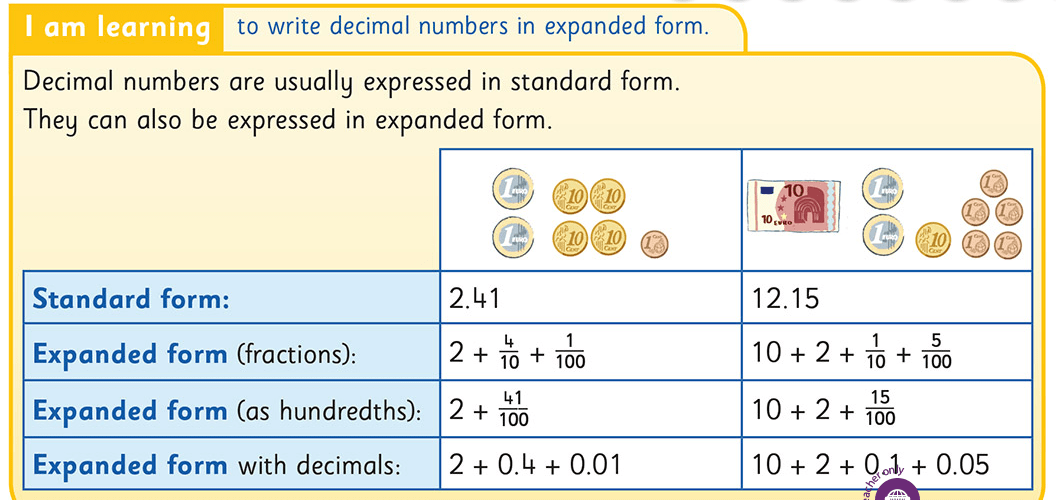However, money is also limited in that it can only be used to represent numbers up to 999.99. This is where the Operation Maths place value discs become extremely useful. Inspired by similar discs used with Singapore Maths, these cut-outs are included in the free ancillary resources that accompany the scheme and can be used in conjunction with the place value mats on the inside back cover of the Discovery Books. With these discs, it is possible to concretely represent numbers up to 99,999, which had not been possible, using resources available in Ireland, prior to the publication of Operation Maths.

When exploring concrete or pictorial representations of numbers, most children will not have much difficulty interpreting the number once it is presented in the typical, canonical arrangement, i.e. 345 as 3H 4T 5U. However, many children may struggle
to interpret the number correctly if it is presented in a non-canonical arrangement, e.g. 345 as 3H 3T 15U or as 2H 14T 5U. Including activities based on these less common, non-canonical arrangements can encourage children to better understand the relationship between the places and can allow you to better assess the depth of the children’s conceptual understanding, while also preparing them for regrouping.

Operation Maths users can also use the excellent Place Value e-Manipulative, accessible on www.edcolearning.ie. This manipulative can be used to show blocks, straws, money or discs to represent a variety of numbers up to 99,999 and to two decimal places.

2. Click on the Pupil Book icon for your class level.
3. Click on the Edco Resources icon (on book cover image on left-hand side)
4. Select e-Manipulatives from list of categories and then Place Value e-Manipulative.

Read also this post from Beyond Tradition Math showing children representing three-digit numbers in various ways. And watch this video from Origo One on using Numeral Expanders to show expanded form.

### Whole Numbers and Decimal Numbers

Since whole number place value and decimal place value are inherently linked, in Operation Maths for 5th and 6th classes, in the topic of place value the children will explore both whole number and decimal place value together in a very holistic way, thus reinforcing their connectedness, within this strand unit.

While place value understanding includes both whole and decimal numbers, it is important that the children appreciate the differences between them. For example, whole numbers and decimal numbers differ in the variety of correct ways in which they can be written. One ten in standard form is usually written as 10; however, one tenth can be written as 0.1, .1, 0.10, 0.100, etc. For decimals, many teachers often only use one form, usually 0.1, fearing that a variety of ways may confuse children. Conversely, using a variety of ways can actually help reinforce children’s understanding that all of the above forms show one tenth (i.e. a 1 in the tenth place immediately to the right of the decimal point), with most forms (excluding .1) having unnecessary zeros (i.e. in 0.3 the zero is unnecessary; without it the value is still 3 tenths). In other numbers, zero acts as a necessary placeholder between the digits in the neighbouring places; in 30 and .304 the zeros are necessary: without them the values would be 3 units and .34 respectively.

### Verbalising Numbers

For most of our number system, we read numbers in the order that we see the digits, e.g. 345 is three hundred and forty-five. However, the numbers from 11 to 19 are an exception, and as such can present extra difficulties for struggling children. Even for the child who begins to appreciate the meaning of ‘-teen’ as ‘and ten’, the numbers 11 and 12 are additional exceptions to this pattern. Some children may also have difficulties with the -ty numbers (e.g. 120, 130, 140) and in particular may confuse them with the similar -teen number, especially in its verbal form, e.g. fifty vs. fifteen. Even children in the middle and senior classes can struggle to distinguish between the -teen and -ty numbers and it is worth being aware of them as potential hurdles, during this topic.

Regarding decimal numbers, the children can use both decimal language and/or fractional language, i.e. expressing 7.381 as seven point three eight one and also seven and three hundred and eighty one thousandths. Using fractional language to read decimals reinforces the value of the digit(s) in the decimal place(s). However, when using decimal language, it is incorrect to say ‘seven point three hundred and eighty one’, as this refers to hundreds and tens (–ty) which are both whole numbers.

### Number Sense and Visualisation Skills

What is bigger; 12.352 or 12.952? When ordering or comparing, children may use a procedure of comparing digits, which involves examining the digits and realising that both numbers have 1 ten, both have 2 units and the first has only 3 tenths, while the second has 9 tenths, so it is bigger. While this procedure may work successfully, it does not encourage the child to visualise the quantities involved. It would be better for the child to recognise that 12.952 is almost 13 and 12.352 is only a little more than 12 and is therefore smaller. Similarly, with younger classes, it is better for the child to recognise that 52 is just a little more than 50 whereas 58 is almost 60. Asking the children to place the numbers on an empty number line (see below) is an ideal way to promote the development of these visualisation skills. Empty number lines are also a great visual strategy to use when rounding; when rounding 12.352 to the nearest unit we can see that it is between 12.3 and 12.4 so it is closer to 12. The children can use the partial number lines in their Discovery Books as an introduction to this method and then be encouraged to solve the rounding activities in their Pupils’ Books by drawing their own number lines, either on their MWBs or in their copies.When considering rounding, it it worth noting that it is preferable to use the phrase ‘round(s) to’ as opposed to ‘round(s) up’ and ‘round(s) down’ as these can confuse many children. For example, some people may say the number 69 rounds up to 70, which makes sense since the digit in the tens place has gone up from 6 to 7. However, if a child realises that 43 should be rounded down, they might change it to 30 instead of 40, since that looks more correct to them, i.e. the tens digit has gone down to 3.

For another idea on how to use number lines to aid rounding, please check out this short video from Origo OneSome traditional tasks and activities for place value regularly seen in maths books can give an incorrect picture of a child’s understanding of the concepts of place value. For example, tasks that involve no more than the children identifying the number of identical dots on a notation board, or the number of identical beads on a place value abacus (see opposite), are not good indicators of a child’s understanding of place value, as they are simply demonstrating their number knowledge of numbers to 9. Therefore, these types of tasks have not been included in the Operation Maths series.

### Bigger Numbers

It is vital the children realise that the digits in larger numbers are organised in groups of three with commas as the digit group separators. Insist that the children also use commas in this way, and reiterate that if you can read a three-digit number you can read any size number as long as there are commas present and that you understand the role of the different commas (i.e. one comma means thousands, two shows millions etc.).Interestingly, using commas as digit group separators is a convention largely in English-speaking countries , and other European countries tend to use stops/point or spaces. It is a good idea to highlight this to the older classes, as is done in Operation Maths 6.

It is also worth noting that the concept of the size of a thousand or the size of a million is in itself quite abstract for children. When tackling the large numbers, use all available opportunities to make them real and relevant to children. There is a Maths Around Us video available in the digital resources of Operation Maths 6 made for this purpose; just click on the hyperlink when accessing the digital book.Also worth noting, is that Operation Maths explores numbers up to 5 digits (whole numbers) in 5th class and through millions in 6th class. However, in the curriculum there is no number limits in 5th and 6th class, therefore the children should not necessarily be limited to these numbers, particularly if they encounter bigger numbers in their environment, books, media, etc. The content in Operation Maths for these classes is deliberately presented in such a way as to encourage children to address numbers above millions where appropriate.

### Place Value in the Environment

As mentioned mentioned previously, it is very important that the children can relate their understanding of place value to numbers around them. To reinforce the relevance of place value, ask the children to collect examples of numbers from the environment. This could include photographs of numbers in the school grounds or locality e.g. car registration numbers, distances on road signs (for Operation Maths 3 users, check out the Maths Trail in the car park, on page 4 of the Discovery Book) . It could also include examples of numbers from print media e.g. newspapers, magazines. In the older classes, you could challenge the children to find an example of a very large number and/or one with the most places of decimals (Operation Maths 6 users, check out the Maths Trail on the internet, on page 6 of the Discovery Book).

What to do with the numbers that the children locate:

• Make a display for the classroom with the examples, in order of size, and giving information for the fact it relates to e.g. the distance to the nearest town etc
• For each example the children find, they must write out the number in word and expanded form (it will likely be in standard form)
• Round the number to the biggest place i.e. if the number is 312 round it to the hundreds, if it’s 0.012 round it to the hundredths etc.